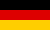# Bri's worldelectronics, programming and more

deutsch# Control theory: control loop PID tuning with oscillation (relay method)

For a stable control loop the control parameters have to be set that no oscillations will occur. There are several methods to estimate the parameters for a stable control loop. In this application I use the relay or oscillation method.

This methods uses a switch to stimulate the control path for oscillations. The measured amplitude and oscillation period is used to estimate the PID controller parameters. A requirement for this method is that the control path can perform oscillations. Most control paths containing a dead time control element are able to oscillate. If the control path is not able to oscillate the PID controller parameters may be estimated with the step response method.

## Control loop

First you have to define the control loop. This is done by selecting the control element types in the table below. The control elements time and gain parameters can be changed in the columns next to the control element type. The Start button is used to draw the control loop step response.

The control loop is stimulated to oscillate at its operating point. The comparator is used to switch the control path input between umin and umax. If the output is greater than the operating point the input will be set to umin. If the output is lesser than the operating point the input will be set to umax.

## Comparator/relay parameters

Controller Operating point Limit u min Limit u max
Comparator

## Control loop parameters

Number Control element Parameter K Parameter T Parameter D Transfer function
1
2
3
4

## Control path parameter calculation

The amplitude and oscillation period are measured from the oscillation. These two parameters are used to calculate the critical gain and the critical period.

\begin{align} P_U& = P\\ K_U& = \frac{4 \cdot A_U}{\pi \cdot A_Y} \end{align}
Parameter Beschreibung Wert
AU Control path input amplitude
AY Control path output amplitude
P Oscillation period
KU Critical gain
PU Critical period

## PID controller parameter calculation

The critical gain and the ciritcal period are used to calculate the PID controller parameters.

Specification Kp Ki Tn = Kp / Ki Kd Tv = Kd / Kp
Ziegler/Nichols $$K_P = 0.6 \cdot K_U; K_I = \frac{2 \cdot K_P}{P_U}; K_D = \frac{K_P \cdot P_U}{8}$$
less overshot $$K_P = 0.33 \cdot K_U; K_I = \frac{2 \cdot K_P}{P_U}; K_D = \frac{K_P \cdot P_U}{3}$$
no overshot $$K_P = 0.2 \cdot K_U; K_I = \frac{2 \cdot K_P}{P_U}; K_D = \frac{K_P \cdot P_U}{3}$$

## Control loop step response

### Simulation parameters

 Step height Step time Simulation end time

### PID controller parameters

 PID Parameter Ziegler/Nichols less overshot no overshot Kp Ki Kd Limit u min Limit u max